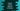# Python 3 program to convert a decimal number to ternary (base 3)## Introduction :

In this python programming tutorial, we will learn how to convert a decimal number to a ternary number.

The decimal number system uses 10 as the base and it has 10 different numerals to represent a number : 0, 1, 2, 3, 4, 5, 6, 7, 8, and 9. We use these nine digits to represent any number in the decimal number system.

Ternary numbers are base 3 numbers. These numbers have three as the base. Three different numerals are used to represent a number in Ternary system: 0,1 and 2. All numbers are represented by using only these three digits.

We can convert any decimal number to its ternary equivalent. For example,

``````Ternary representation of decimal 0 is 0.
Ternary representation of decimal 1 is 1.
Ternary representation of decimal 2 is 2.
Ternary representation of decimal 3 is 10.
Ternary representation of decimal 4 is 11.
Ternary representation of decimal 5 is 12.
Ternary representation of decimal 6 is 20.
Ternary representation of decimal 7 is 21.
Ternary representation of decimal 8 is 22.
Ternary representation of decimal 9 is 100.
Ternary representation of decimal 10 is 101 etc.``````

In this tutorial, we will learn how to convert a decimal number to its ternary representation programmatically using python.

Before start writing the program, let’s try to understand how the conversion works :

• Suppose we want to convert 21(decimal) to ternary.
• Divide 21 by 3, remainder is 0, quotient is 7
• Divide 7 by 3, remainder is 1, quotient is 2
• Divide 2 by 3, remainder is 2, quotient is 0

Now, append all remainders 2-1-0, that’s it. 210 is the conversion. So, keep dividing the number by 3 till the quotient is 0 and append all remainders in reverse order. Easy, isn’t it?

Using the steps shown above, we can easily implement the algorithm in any programming language. Let’s take a look at the python program to convert decimal to ternary in python

(The below example program is available here on Github) :

### Python program to convert a decimal number to ternary :

``````def find_ternary(num):  #2
quotient = num/3    #3
remainder = num%3
if quotient == 0:   #4
return ""
else:
return find_ternary(int(quotient)) + str(int(remainder))    #5
number = int(input("Enter a number : ")) #1
print(find_ternary(number))``````

### Explanation :

The commented numbers in the above program denote the step number below :

1. First, ask the user to enter a number. Read the number and store it in number variable.
2. findternary_ method is a recursive method that is used to find out the ternary value recursively.
3. First of all, it will find out the quotient and remainder of the given number.
4. If the quotient is 0, it will return one empty string. Else, it will call itself recursively by passing the quotient as an argument and appending the remainder to it. Basically, it is doing the same thing that we have seen in the example above.

### Sample Output :

In this program, we are asking the user to enter the decimal value and we are calculating the ternary representation for that value recursively. The recursive process is useful if we need to perform a similar operation continuously. Here, we are continuously dividing the number by 3 until the quotient becomes 0. Never forget to add a condition to check for the end in a recursive function. Or else it will keep running for infinite time.

### Conclusion :

In this tutorial, we have learned about the ternary number system and how to convert a decimal number to ternary using python. Go through the example above and try to run the program using python 3. If you have any queries, drop one comment below.